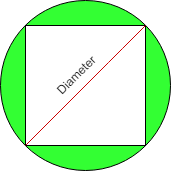# Area of square Circumscribed by Circle

• Last Updated : 22 Jun, 2022

Given the radius(r) of circle then find the area of square which is Circumscribed by circle.
Examples:

```Input : r = 3
Output :Area of square = 18

Input :r = 6
Output :Area of square = 72```

All four sides of a square are of equal length and all four angles are 90 degree. The circle is circumscribed on a given square shown by a shaded region in the below diagram.Properties of Circumscribed circle are as follows:

• The center of the circumcircle is the point where the two diagonals of a square meet.
• Circumscribed circle of a square is made through the four vertices of a square.
• The radius of a circumcircle of a square is equal to the radius of a square.

Formula used to calculate the area of circumscribed square is:
2 * r2
where, r is the radius of the circle in which a square is circumscribed by circle.
How does this formula work?
Assume diagonal of square is d and length of side is a.
We know from the Pythagoras Theorem, the diagonal of a
square is √(2) times the length of a side.
i.e d2 = a2 + a2
d = 2 * a2
d = √(2) * a
Now,
a = d / √2
and We know diagonal of square that are Circumscribed by
Circle is equal to Diameter of circle.
so Area of square = a * a
= d / √(2) * d / √(2)
= d2/ 2
= ( 2 * r )2/ 2 ( We know d = 2 * r )
= 2 * r2

## CPP

 `// C++ program to find Area of``// square Circumscribed by Circle``#include ``using` `namespace` `std;` `// Function to find area of square``int` `find_Area(``int` `r)``{``    ``return` `(2 * r * r);``}` `// Driver code``int` `main()``{``    ``// Radius of a circle``    ``int` `r = 3;``    ` `    ``// Call Function to find``    ``// an area of square``    ``cout << ``" Area of square = "``                 ``<< find_Area(r);``    ` `    ``return` `0;``}`

## Java

 `// Java program to find Area of``// square Circumscribed by Circle``class` `GFG {``    ` `    ``// Function to find area of square``    ``static` `int` `find_Area(``int` `r)``    ``{``        ``return` `(``2` `* r * r);``    ``}``    ` `    ``// Driver code``    ``public` `static` `void` `main(String[] args)``    ``{``        ``// Radius of a circle``        ``int` `r = ``3``;` `        ``// Call Function to find``        ``// an area of square``        ``System.out.print(``" Area of square = "``                             ``+ find_Area(r));``    ``}``}` `// This code is contributed by Anant Agarwal.`

## Python3

 `# Python program to``# find Area of``# square Circumscribed``# by Circle` `# Function to find``# area of square``def` `find_Area(r):` `    ``return` `(``2` `*` `r ``*` `r)``    ` `# driver code``# Radius of a circle``r ``=` `3``    ` `# Call Function to find``# an area of square``print``(``" Area of square = "``, find_Area(r))` `# This code is contributed``# by Anant Agarwal.`

## C#

 `// C# program to find Area of``// square Circumscribed by Circle``using` `System;` `class` `GFG {``    ` `    ``// Function to find area of square``    ``static` `int` `find_Area(``int` `r)``    ``{``        ``return` `(2 * r * r);``    ``}``    ` `    ``// Driver code``    ``public` `static` `void` `Main()``    ``{``        ``// Radius of a circle``        ``int` `r = 3;` `        ``// Call Function to find``        ``// an area of square``        ``Console.WriteLine(``" Area of square = "``                            ``+ find_Area(r));``    ``}``}` `// This code is contributed by vt_m.`

## PHP

 ``

## Javascript

 ``

Output:

`Area of square = 18`

Time Complexity: O(1)
Auxiliary Space: O(1)

Please suggest if someone has a better solution which is more efficient in terms of space and time.